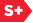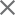### Proceedings Paper

Adaptive self-quantization of wavelet subtrees: a wavelet-based theory of fractal image compression
Author(s): Geoffrey M. Davis
Format Member Price Non-Member Price
PDF \$17.00 \$21.00

Paper Abstract

Fractal image compression was one of the earliest compression schemes to take advantage of image redundancy in scale. The theory of iterated function systems motivates a broad class of fractal schemes but does not give much guidance for implementation. Fractal compression schemes do not fit into the standard transform coder paradigm and have proven difficult to analyze. We introduce a wavelet-based framework for analyzing fractal block coders which simplifies these schemes considerably. Using this framework we find that fractal block coders are Haar wavelet subtree quantization schemes, and we thereby place fractal schemes in the context of conventional transform coders. We show that the central mechanism of fractal schemes is an extrapolation of fine-scale Haar wavelet coefficients from coarse-scale coefficients. We use this insight to derive a wavelet-based analog of fractal compression, the self-quantization of subtrees (SQS) scheme. We obtain a simple SQS decoder convergence proof and a fast SQS decoding algorithm which simplify and generalize existing fractal compression results. We describe an adaptive SQS compression scheme which outperforms the best fractal schemes in the literature by roughly 1 dB in PSNR across a broad range of compression ratios and which has performance comparable to some of the best conventional wavelet subtree quantization schemes.

Paper Details

Date Published: 1 September 1995
PDF: 13 pages
Proc. SPIE 2569, Wavelet Applications in Signal and Image Processing III, (1 September 1995); doi: 10.1117/12.217585
Show Author Affiliations
Geoffrey M. Davis, Dartmouth College (United States)

Published in SPIE Proceedings Vol. 2569:
Wavelet Applications in Signal and Image Processing III
Andrew F. Laine; Michael A. Unser, Editor(s)